Published: 15 August 2015

# Investigation on forced vibration of turbocharger turbine in pulsation flow

Yixiong Liu1
Dazhong Lao2
Ce Yang3
1, 3School of Mechanical Engineering, Beijing Institute of Technology, Beijing 100081, China
2School of Aerospace Engineering, Beijing Institute of Technology, Beijing 100081, China
Corresponding Author:
Dazhong Lao
Views 805

#### Abstract

Variable guide vanes turbines are widely applied in turbocharger. To assess the effects of pulsation inlet conditions on the aerodynamic excitation and the forced vibration of turbine wheel, the weak fluid structure interaction method was adopted. Firstly, a pulsation flow with the frequency of 60 Hz (which corresponds to the engine speed of 2400 r/min) was applied at the turbine inlet to conduct the unsteady flow calculation. The results show that the aerodynamic loads are highly influenced by the pulsation inlet conditions, which fluctuate with the inlet pulsation wave in a pulse cycle, and the maximum and minimum blade load corresponding to the peak and trough of the inlet pulsation wave respectively. For the forced vibration analysis of turbine wheel, the calculated aerodynamic loads in pulsation flow were imposed as the loading boundaries to perform the harmonic vibration and transient structural response by FE calculations, based on the natural frequency and mode of the blade disk. The results show that the forced response of the blade disk not only reflects the contribution of low frequency pulsation excitations but also contains the high frequency pressure fluctuations caused by rotor rotation.

## 1. Introduction

Nowadays, most vehicle engines are turbocharged to meet the ever-increasing demands of stricter exhaust emission, higher engine power and fuel economy. Compared with the heavy turbomachines applied to the power plants, the exhaust gas turbochargers used in the automobiles are much smaller and work at higher rotor speeds in various operating conditions. The rotor speeds of turbine and compressor wheels are up to 120,000 rpm and the maximum circumferential velocity of turbine is approximately 560 m/s . The aerodynamic and inertial forces acting on the rotor blades will cause excessive rotor forced vibration and lead to structural high cycle fatigue (HCF) [2, 3]. Therefore, the forced response of the turbocharger turbine caused by the aerodynamic excitations should be paid special attentions.

Additionally, for the blade disk of turbine, mistuning inevitably exits due to small differences in geometry and material among the blades, which causes the number of possible resonances increase significantly and leads to mode localization and induces significant dynamic stress [12-14]. Researches on mistuning identifications have been performed mainly in the aero engine fields. Recently, mistuning investigations are gradually conducted in automotive turbocharger. Hemberger et al. [15-17] pointed out that the mistuning of turbine or compressor leads to an amplification of blade vibration amplitudes and hence to increases the stresses of blades by theoretical and experimental investigations. And the numerical models of the mistuning effects of turbine wheels for automotive turbocharger applications were created and validated. Hattori  investigated the mistuned vibration for the turbine blade disk of automotive turbocharger considering the frequency and geometric mistuning.

In this work, the non-uniform pulsation flow through the turbine was investigated and the pressure perturbation caused by the pulsation flow was obtained. The application of numerical method based on weak fluid and structure interaction (FSI) was adopted to evaluate the blade vibration and forced response of the turbine wheel with the effect of mistuning issue considered.

## 2. Theory analysis

The partially coupling method was used to solve the coupled fluid and structure problems for turbocharger turbine. The sequential coupling method introduced by Filsinger et al. [19, 20] was adopted, which based on the assumption of the sufficient small blade deformation so that aerodynamic excitation can be calculated by assuming to a non-vibrating structure.

For the unsteady flow calculation, the Reynolds-Averaged Navier-Stokes (RANS) equations are expressed by the form of integral as follow:

1
$\frac{\partial }{\partial t}\underset{\mathrm{\Omega }}{\int }\mathbf{U}d\mathrm{\Omega }+\underset{S}{\int }{\mathbf{F}}_{I}dS+\underset{S}{\int }{\mathbf{F}}_{V}dS=\underset{\Omega }{\int }{\mathbf{S}}_{T}d\mathrm{\Omega },$

where, $\mathrm{\Omega }$ is the volume and $S$ is the surface; $\mathbf{U}$ is the vector of the conservative variable; ${\mathbf{F}}_{I}$ and ${\mathbf{F}}_{V}$ are respectively the inviscid and viscous flux vectors; ${\mathbf{S}}_{T}$ contains the source terms.

By numerical solving the RANS equations, the time-resolved pressures on the blade surfaces are obtained, which can be divided into two parts, including the time average term and the fluctuation term, given by:

2
$p\left(r,\theta ,z,t\right)=\overline{p}\left(r,\theta ,z\right)+\stackrel{^}{p}\left(r,\theta ,z,t\right).$

For the pressure fluctuation term, it is time-variation. The Fourier transformation (FFT) is performed to convert the pressure fluctuation from the time domain to frequency domain.

The general structural dynamic equations are written as follow:

3
$\left[\mathbf{M}+\mathrm{\Delta }\mathbf{M}\right]\left\{\stackrel{¨}{u}\right\}+\left[{C}_{m}+{C}_{aero}\right]\left\{\stackrel{˙}{u}\right\}+\left[\mathbf{K}+\mathrm{\Delta }\mathbf{K}\right]\left\{u\right\}=\left\{\mathbf{F}\right\},$

where, $\mathbf{K}$ and $\mathbf{M}$ are the stiffness and mass matrix; ${C}_{m}$ and ${C}_{aero}$ are mechanical and aerodynamic damping matrix; $u$ is the displacement, and $F$ is the force. Deviations of the mass $\mathrm{\Delta }\mathbf{M}$ and stiffness $\mathrm{\Delta }\mathbf{K}$ respectively represent the mass and frequency mistuning.

To model the mistuned systems of the turbocharger turbine blade disk, a reduced order model is used, which has been detailed discussed in ref. [21, 22]. By assuming the aerodynamic excitation as the sinusoidal disturbance, the steady-state harmonic responses of mistuned blade disk are conducted. The mode superposition method is adopted to solve the structural dynamic equations, which uses the natural frequencies and mode shapes to solve the response of a sinusoidal varying forcing function. Convert the motion equations to modal form:

4
$\left\{{\stackrel{¨}{y}}_{j}\right\}+2{f}_{j}{\xi }_{j}{\left\{\stackrel{˙}{y}}_{j}\right\}+{f}_{j}^{2}\left\{{y}_{j}\right\}=\left\{{p}_{j}\right\},$

where, the mistuning effects in Eq. (3) are all considered. ${y}_{j}$ is the modal coordinate, ${f}_{j}$ is natural circular frequency, ${\xi }_{j}$ is ratio of critical damping, ${p}_{j}$ is pressure in modal coordinate.

For a steady sinusoidal vibration, ${y}_{j}$ and ${p}_{j}$ have the forms:

5
${y}_{j}={y}_{jc}{e}^{i\omega t},{p}_{j}={p}_{jc}{e}^{i\omega t},$

where, ${y}_{jc}$ is complex amplitude of modal coordinate, ${p}_{jc}$ is complex force amplitude, $\omega$ is imposed circular frequency. Here, the pressure ${p}_{j}$ is assumed as the harmonic form because the inlet pulsation is simplified by the sinusoidal, and the pressure fluctuation is similar to the sinusoidal harmonic form.

Substituting Eq. (5) into Eq. (4), the complex amplitude of modal coordinate is obtained:

6
${y}_{jc}=\frac{{p}_{jc}}{\left({f}_{j}^{2}-{\omega }^{2}\right)+i\left(2{f}_{j}\omega {\xi }_{j}\right)}.$

The complex displacements are obtained:

7
$\left\{{u}_{c}\right\}=\sum _{j=1}^{n}\left\{{\phi }_{j}\right\}{y}_{jc},$

where, ${u}_{c}$ is complex displacements, ${\phi }_{j}$ is the mode shape.

After providing all the input parameters and solving the fluid and structural equations, the transient pressure fluctuations on blade surfaces and the forced response characteristics of turbine blade disk are obtained.

## 3. Calculation models and boundaries

In this work, the JK90S turbocharger is adopted, which fixes to a 6-cylinder diesel engine, the assembly drawing of the turbocharger is shown in Fig. 1(a). The rated speed of the engine is 2400 r/min, corresponding to the pulsation frequency of 60 Hz. In this paper, the turbine stage is investigated, marking with the red dotted line regions in Fig. 1(a), including volute, guide vanes and rotor blades. For the rotor, the numbers of blades are 10, the inlet diameter is 83.5 mm, the outlet diameter is 73.5 mm, and the endwall clearance is 0.6 mm. For the nozzle, the numbers of vanes are 15 and the endwall clearance is 0.6 mm.

Considering the time-consuming and computing resources of the unsteady calculation with pulsation inlet conditions, the numerical model of turbine was simplified and the volute was neglected in CFD simulations, and the numbers of the rotor blade and nozzle vane were reduced to 2 and 3 respectively according to the blade reduction method of turbomachinery. The structured hexahedron multi-block topology was adopted to generate computation grids by numerical software. As shown in Fig. 1(b), the girds of single nozzle passage were about 150,000 and the numbers of single rotor blade passage grids were almost 250,000. The total grids numbers of turbine computation domain were about 1,000,000.

The material of impeller is chrome-nickel alloy, the density $\rho =$ 8000 kg/m3, the Poisson ratio $\mu =$ 0.3, the elastic modulus $E=$ 2×105 MPa, the ultimate yield strength ${\sigma }_{s}=$760 MPa. Solid187 element is used to generate the FE calculation grids, which has three degrees of freedom at each node: translations in the nodal $x$, $y$, and $z$ directions. The element has plasticity, stress stiffening, large deflection and large strain capabilities; it also has a quadratic displacement behavior and is well suited to model irregular meshes. The FE grids of the turbine were shown in Fig. 1(c). It was consisted of almost 90,000 tetrahedron elements. A modal eigen-value extraction of Block Lanczos method was performed to obtain the eigen-frequencies and mode shapes. In dynamic calculations, the turbine wheel shaft was cylindrical supported to load the rotational speed, the radial and axial direction were fixed, the tangential was free, and blades were fixed on the hub.

According to the test results of pulsation exhaust conducted by Dale and Watson , as shown in Fig. 2. It can be observed that the pressures and temperatures of the engine exhaust were changing with time, and the pulsation wave was similar to the sinusoid curve.

Fig. 1Models of turbine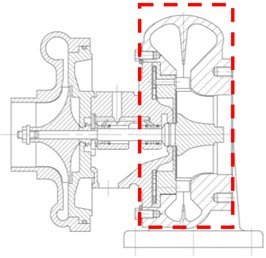a) Turbocharger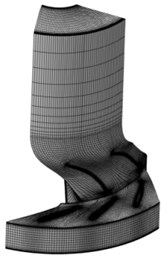b) CFD grids of turbine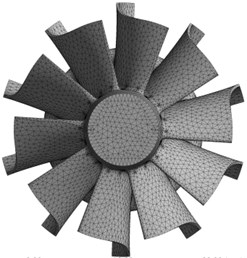c) FEA grids of turbine

Fig. 2Pulsation exhaust features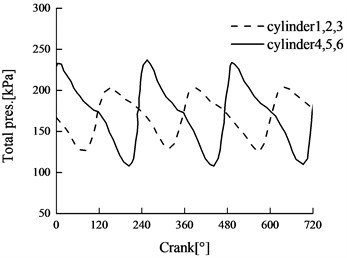a) Total pressure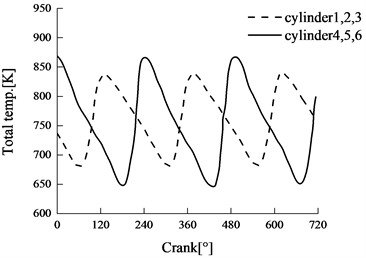b) Total temperature

In this work, the pulsating total pressure and total temperature were simulated by sinusoidal variation curves of total pressure/temperature and imposed at turbine inlet for the CFD calculations, as follow:

8
${h}_{t}={h}_{a}-A\mathrm{s}\mathrm{i}\mathrm{n}\left(2\pi \omega t\right),$

where, ${h}_{t}$ is transient total value, ${h}_{a}$ is the average value in pulsation cycle, $A$ is the amplitude, $\omega$ is the frequency. Here, the pulsation frequency is determined by the number of cylinders of the engine rotational speed.

As illustrated in Fig. 3, the solutions of the fluid and structural equations are performed by the decoupled method. The unsteady flow of the turbine with pulsating inlet conditions, discussed above, is conducted by solving the RANS equations. The CFD analysis is performed not only to obtain the aerodynamic excitations due to unsteady interaction of pulsating flow in turbine internal, but also to get the aerodynamic damping ratio. Then, the aerodynamic loads are transformed from time domain to frequency domain by FFT. Harmonic vibration of the turbine is performed, with considering the aerodynamic excitations provided by periodic aerodynamic disturbances of pulsating flow, aerodynamic and mechanical damping and the mistuning issue of the blade disk. The natural frequencies and mode shapes of the turbine blade disk are obtained by modal analysis using the commercial FE solver. The forced response analysis including the harmonic vibration and transient structural response are conducted.

Fig. 3A schematic diagram of solution process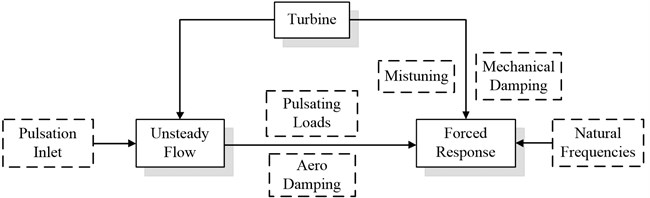## 4. Results and discussion

The unsteady flow calculation of the turbine under pulsation inlet condition was performed using the numerical models and boundaries presented above. The numerical calculation process consumed more than 100 CPU time with 8 nodes parallel computation and took up more than 300 GB computer memory. Considering the numerical convergence criterion, the mass flows at inlet and outlet were monitored, and the difference is 1.485 %, which can be acceptable .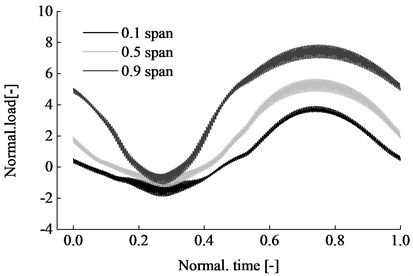Fig. 5Pulsation inlet conditions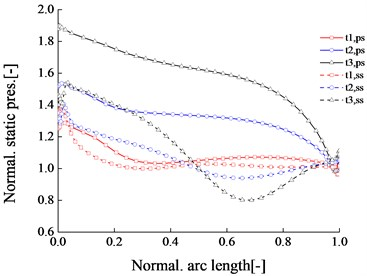Fig. 5 shows the static pressures on the PS and SS at various typical pulsation time steps. In the figure, $t1$, $t2$, $t3$ correspond to the wave trough, the equilibrium position and the wave peak of the pulsation inlet respectively. It can be seen that the pressure difference between PS and SS side at $t1$ is the minimum, at $t3$ is the maximum and at $t2$ is the middle, which corresponds to the trough and peak locations of the inlet pulsation waves.

The FFT is conducted to transfer the blade loading from time-domain to frequency domain. The result of FFT contains the frequency data and the complex transformed results. Meanwhile, it can also provide the magnitude, amplitude, phase, power density and other computation results. The power density estimation can be made by three different methods: MSA, SSA and TISA. Fig. 6 and Fig. 7 shows the FFT results of blade loadings at 0.5 span, including the amplitude-frequency characteristics and MSA power density features. It can be seen that the low frequency characteristics of the blade loading are mainly reflected by the inlet pulsating frequency, especially for the one time and twice of the pulsating frequency. The power spectrum curve also shows the dominant role of the integral multiple of the pulsating frequency.

Fig. 6Amplitude-frequency characteristic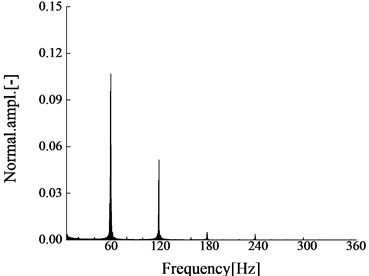Fig. 7Power-frequency diagram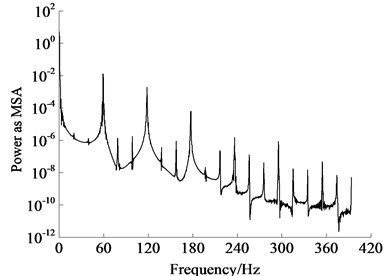### 4.2. Natural frequencies and modes

The finite element analysis of turbine rotor were performed to extract the modal eigenvalues and to obtain the structure’s vibration behaviors. For the coupled blade disk of turbine, the vibration modes can be characterized by the node diameter lines of turbine wheel. Nodal diameter (ND) is used to describe the vibration characteristic of blade disk, and the detailed description can be referred by refs. [9, 11].

Fig. 8Mode shapes of blade disk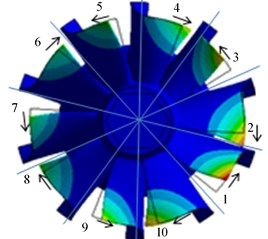a) ND 5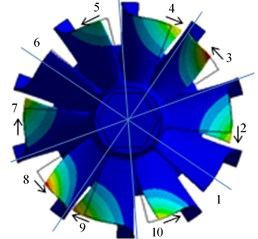b) ND 4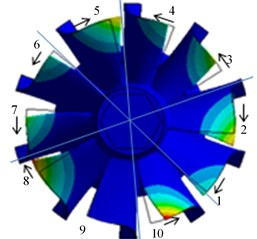c) ND 3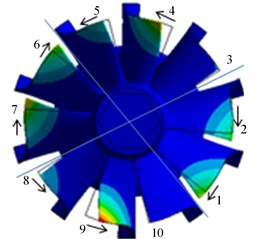d) ND 2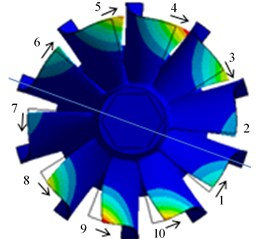e) ND 1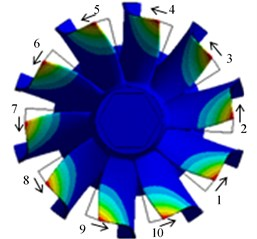f) ND 0

Fig. 9Blade tips deformations in various NDs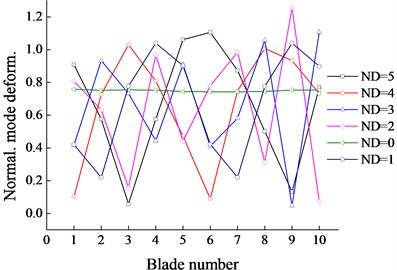Fig. 10First natural frequencies in various NDs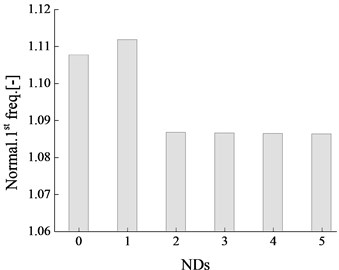### 4.3. Forced vibrations

Fig. 11Blade tips deformations in various NDs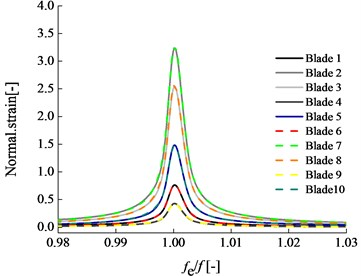Fig. 12First natural frequency in various NDs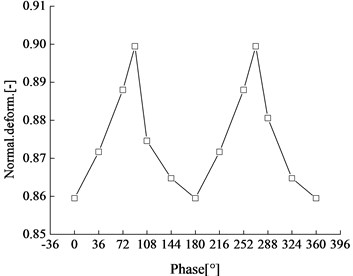Fig. 13Transient deformations in a pulsation cycle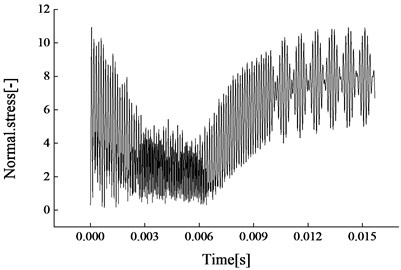Fig. 14Transient stresses in a pulsation cycle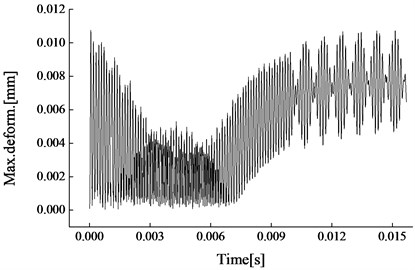Further, the transient structural response of the blade disk is performed after putting the aerodynamic excitations and measured damping, combining with the natural frequency and mode. The deformations and the stresses of the blade disk in a pulsation cycle are presented in Fig. 13 and 14. It can be seen that both the transient deformations and the stresses and are changing with the pulsating pressure fluctuations. In a pulsation cycle, the deformations and the stresses of the blade disk are following by the pulsating wave of inlet condition. Meanwhile, the high frequency rotational characteristics of the turbine rotor are also reflected in the figures. The deformations and stresses are jumping in a rotor rotational cycle, and the time is shorter compared with pulsation steps. Therefore, the forced vibration of the blade disk in pulsation flow is not only affected by the low frequency pulsating excitation, but also affected by the high frequency excitation of rotor rotation due to the rotor-stator interaction, wake and potential flow.

## 5. Conclusions

The work in this paper investigated the aerodynamic excitation and the forced vibration of a automotive turbocharger turbine with the variable guide vanes under pulsation inlet conditions. A pulsation flow with the frequency of 60 Hz was applied at the turbine inlet according to the exhaust of the engine and the unsteady calculation of the turbine was performed. The calculation process consumed more than 100 CPU time with 8 nodes parallel computations and took up more than 300 GB computer memory. The results of the unsteady flow in turbine internal demonstrated the aerodynamic loads of the blades are highly influenced by the pulsation inlet conditions, which follow the change of the inlet pulsation wave, in a pulse cycle, the maximum and minimum corresponding to the peak and trough of the pulsation inlet respectively.

A detailed analysis for the natural dynamic behaviors of the turbine wheel was conducted, especially, the first order natural mode was paid special attentions. The results of the eigen mode of the blade disk show that nodal diameter vibrations appear in pairs, marked with ND 0, ND 1, ND 2, ND 3, ND 4 and ND 5. Compared with ND 0, the vibration modes in other NDs are separated by their ND lines, and the deformations of the natural vibration are various. The phases among the blades significantly affect the vibration of turbine blade disk. Blades close to the nodal points on the disk bear the maximum excitations accompanying with the maximum blade tip deformations, whereas blades at the antinodes are much less or even stand still. The maximum stress caused by the first order vibration locates at the trailing edge of the blade.

28 April 2015
Accepted
28 July 2015
Published
15 August 2015
SUBJECTS
Flow induced structural vibrations
Keywords
turbocharger
turbine
pulsation flow
forced vibration
Acknowledgements

The work was funded by National Natural Science Foundation of China (No. 51276017).